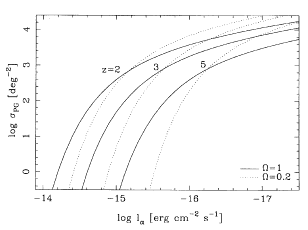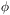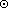### 3. MODELS OF PRIMEVAL GALAXIES

The empirically derived properties of PGs discussed in Section 2 can be compared with the predictions of several models. One of the first ``modern'' PG models was that of Meier (1976); this model was based on the hydrodynamic collapse computations of Larson (1974). Because of strong dissipation in these models, the prediction of Meier was that PGs were very compact, with a prominent core/halo structure. Many details of the appearance of PG's were worked out for the first time in this paper.

More recently, BW87 have developed a model for the appearance of PGs in a Universe in which galaxies are dominated by dissipationless, pregalactic dark matter - e.g., CDM (see also Carlberg 1988). They ran an N-body simulation containing both gas and dark matter, assumed a completion of the collapse at around zcoll = 2 (or higher for a bias factor b1), and further assumed a star formation time scale tSFRtcoll, with star formation induced during cloud-cloud collisions. [If tcoll < tSFR, a pure disk system results; whereas if tcoll > tSFR then a pure spheroid results. Hence this assumption is both reasonable and essential. It is interesting to note that the model of Lin and Murray (1992; see below) provides a simple self-regulating mechanism to enforce this near equality of collapse and star formation time scales.]

The result of the BW87 computation was a flattened, lumpy protogalaxy, with ~ 60% of the stars formed in 80% of the collapse time. For a Schechter (1976) luminosity function for present epoch galaxies, the Lyluminosity function is also a Schechter function, with characteristic luminosity L*Ly1043 erg s-1 for a rest-frame Lyequivalent width of 100 Å and collapse time of 1 Gyr (BW87; Pritchet and Hartwick 1990). Figure 5 shows the expected integral Lyluminosity function of PG's for different redshifts of collapse (for a filter bandwidthz = 0.1) (6).Figure 5. The integral Lyluminosity distribution; that is, the surface of Lyemitting objects brighter than a given Lyflux. The models are computed for (top to bottom) z = 2, 3, and 5. Solid lines:0 = 1; dotted lines:0 = 0.2. The model is described in Pritchet and Hartwick (1990), and assumes a collapse time tcoll = 109 yr, spheroid fraction fs = 0.3,* = 0.012 h-2 Mpc-3, and filter bandwidthz = 0.1. The models are not sensitive to the value of H0. Sensitivity of the models to other parameters is explored in Fig. 1 of PH90. (Note that the models in PH90 must be multiplied by a factor of 4in surface density.)

A somewhat similar model has been proposed by Lin and Murray (1992, hereafter LM92). This model is based on a more detailed treatment of star formation: star formation is self-regulated due to the fact that, for much of the PG phase, the cooling or star formation time scale is less than the dynamical time scale. As a result, massive stars will form at a rate just sufficient to keep the ISM of the PG ionized (since ionization and heating of the ISM quenches star formation). The model neglects the gravitational effect of dark matter, and treats galaxy formation as being due to the collapse of a large monolithic perturbation (i.e. it neglects the substructure and mergers that are so important in the BW87 model).

The properties of the LM92 model depend dramatically on the initial configuration of the gas mass. However, a 1011 Mgas cloud that starts off as an isothermal sphere (an initial configuration that might be imposed, for example, by a dark matter halo) maintains a roughly constant luminosity in ionizing photons ~ 1043.5 erg s-1 for roughly 1 Gyr. This corresponds to ~ 1044.5 erg s-1 in bolometric luminosity (cf. Meier 1976). This is almost identical to the luminosity of a 1011 Mbaryonic mass PG predicted by BW87. In addition, the lifetime of the PG phase (~ 1 Gyr) is very similar in the two models.

The principal difference between the BW87 and LM92 models appears to be in appearance: LM92 predicts a strongly centrally concentrated PG, with a smooth, diffuse envelope, whereas the BW87 model naturally predicts a lumpy, extended appearance due to the presence of inhomogeneities and mergers.

6 The models in Fig. 1 of PH90 should be scaled up by a factor of 4in surface density to correct a numerical error. Back.